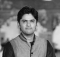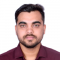## Python Pandas - Filling missing column values with modePublished on 21-Sep-2021 06:51:07
Mode is the value that appears the most in a set of values. Use the fillna() method and set the mode to fill missing columns with mode. At first, let us import the required libraries with their respective aliases −import pandas as pd import numpy as npCreate a DataFrame with ... Read More

## Python Pandas - Fill missing columns values (NaN) with constant valuesPublished on 21-Sep-2021 06:39:52
Use the fillna() method and set a constant value in it for all the missing values using the parameter value. At first, let us import the required libraries with their respective aliases −import pandas as pd import numpy as npCreate a DataFrame with 2 columns. We have set the NaN ... Read More

## Python - Search DataFrame for a specific value with pandasPublished on 21-Sep-2021 06:32:44
We can search DataFrame for a specific value. Use iloc to fetch the required value and display the entire row. At first, import the required library −import pandas as pdCreate a DataFrame with 4 columns −dataFrame = pd.DataFrame({"Car": ['BMW', 'Lexus', 'Tesla', 'Mustang', 'Mercedes', 'Jaguar'], "Cubic_Capacity": [2000, 1800, 1500, 2500, 2200, ... Read More

## Sort index in ascending order – Python PandasPublished on 21-Sep-2021 06:23:07
The sort_index() is used to sort index in ascending and descending order. If you won’t mention any parameter, then index sorts in ascending order.At first, import the required library −import pandas as pdCreate a new DataFrame. It has unsorted indexes −dataFrame = pd.DataFrame([100, 150, 200, 250, 250, 500], index=[4, 8, ... Read More

## How to draw the largest polygon from a set of points in matplotlib?Published on 20-Sep-2021 13:49:14
To draw the largest polygon from a set of points in matplotlib, we can take the following steps −Import "Polygon" from matplotlib.patches.Set the figure size and adjust the padding between and around the subplots.Create a list of data points for the largest polygon.Get the polygon instance.Create a figure and a ... Read More

## How to change the face color of a plot using Matplotlib?Published on 20-Sep-2021 13:32:03
To change the face color of a plot using matplotlib, we can take the following steps −Set the figure size and adjust the padding between and around the subplots.Create x and y data points using numpy.Create a figure and a set of subplots.Plot the x and y data points using ... Read More

## Plotting a masked surface plot using Python, Numpy and MatplotlibPublished on 20-Sep-2021 13:23:51
To plot a masked surface plot using Python, Numpy and Matplotlib, we can take the following steps −Set the figure size and adjust the padding between and around the subplots.Create a new figure or activate an existing figure.Add an 'ax' to the figure as part of a subplot arrangement.Return the ... Read More

## Matplotlib – Drawing lattices and graphs with NetworkxPublished on 20-Sep-2021 13:14:23
To draw lattices and graphs with networkx, we can take the following steps −Import networkx and pyplot.Set the figure size and adjust the padding between and around the subplots.Use nx.grid_2d_graph(3, 3) to get a two-dimensional grid graph. The grid graph has each node connected to its four nearest neighbors.Draw the ... Read More

## How to set the border color of the dots in matplotlib's scatterplots?Published on 20-Sep-2021 13:07:59
To set the border color of the dots in matplotlib scatterplots, we can take the following steps −Set the figure size and adjust the padding between and around the subplots.Initialize a variable "N" to store the number of sample data.Create x and y data points using numpy.Plot the x and ... Read More

## Python - Add a prefix to column names in a Pandas DataFramePublished on 21-Sep-2021 06:16:21
To add a prefix to all the column names, use the add_prefix() method. At first, import the required Pandas library −import pandas as pdCreate a DataFrame with 4 columns −dataFrame = pd.DataFrame({"Car": ['BMW', 'Lexus', 'Tesla', 'Mustang', 'Mercedes', 'Jaguar'], "Cubic_Capacity": [2000, 1800, 1500, 2500, 2200, 3000], "Reg_Price": [7000, 1500, 5000, 8000, ... Read More